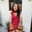Trusted answers to developer questions
Trusted Answers to Developer Questions

Related Tags

# What is LU factorization?Aqsa Amir

Grokking Modern System Design Interview for Engineers & Managers

Ace your System Design Interview and take your career to the next level. Learn to handle the design of applications like Netflix, Quora, Facebook, Uber, and many more in a 45-min interview. Learn the RESHADED framework for architecting web-scale applications by determining requirements, constraints, and assumptions before diving into a step-by-step design process.

### Overview

Breaking the original matrix, $A$ , into an upper triangular matrix, $U$, and a lower triangular matrix, $L$, is known as LU factorization. The product, $LU$should always equal the original matrix, $A$.

The equation can be represented in the matrices as follows:

### Example

The following example depicts the result of LU factorization.

### Method

Let's find the LU factorization of the following example:

Where $A$ is as follows:

### Step 1

Find the upper triangular matrix $U$ using the Gaussian elimination method.

Note: In the Gaussian elimination method,

1. All rows containing zeros must be at the bottom of the matrix.

2. The first non-zero entry of every row should be on the right side of the first non-zero entry of the previous row.

Perform the following operation:

The resulting matrix obtained is as follows:

Now, perform another operation to achieve the upper triangular matrix, $U$:

The resulting matrix obtained is as follows:

### Step 2

#### Method 1

Calculate the lower triangular matrix using the equation $A = LU$, where $L$ is set as an arbitrary matrix and its values will be calculated.

#### Method 2

Otherwise, $L$ can be calculated by equating the arbitrary elements in $L$ to the multiplier coefficients that made those respective elements zero.

We will use the second method to clarify it further.

• $O_{1}$ made $l_{31}$ zero, hence, we will replace it with the multiplier element 2.
• $O_{2}$ made $l_{32}$ zero, hence, we will replace it with the multiplier element -4.
• $l_{21}$ was already zero so it will remain the same.

The resulting matrix is as follows:

### Step 3

• Given that $AX = C$, we will replace $A$ with $LU$ since $A=LU$.
• Now in the equation $LUX=C$, replace $UX$ with $Y$.
• Solve the system of linear equations using the two equations $LY = C$ and $UX=Y$.

Solving the first equation, $LY = C$.

The solution matrix, $Y$, is obtained as follows:

Solving the second equation, $UX=Y$.

The solution matrix, $X$ , is obtained as follows:

Q

Solve the following systems of equations:

6$x_{1}$ + 18$x_{2}$ + 3$x_{3}$ = 3

2$x_{1}$ + 12$x_{2}$ + $x_{3}$ = 19

4$x_{1}$ + 15$x_{2}$ + 3$x_{3}$ = 0

{3, -9, 12}

{1, -3, 7}

{-3, 3, -11}

{-2, -5, 13}

### Applications

LU factorization is used in numerous applications such as:

• Finding the inverse of a matrix
• Finding the determinant of a matrix
• Finding current in a circuit
• Solving discrete dynamical system problems

RELATED TAGS

CONTRIBUTORAqsa Amir